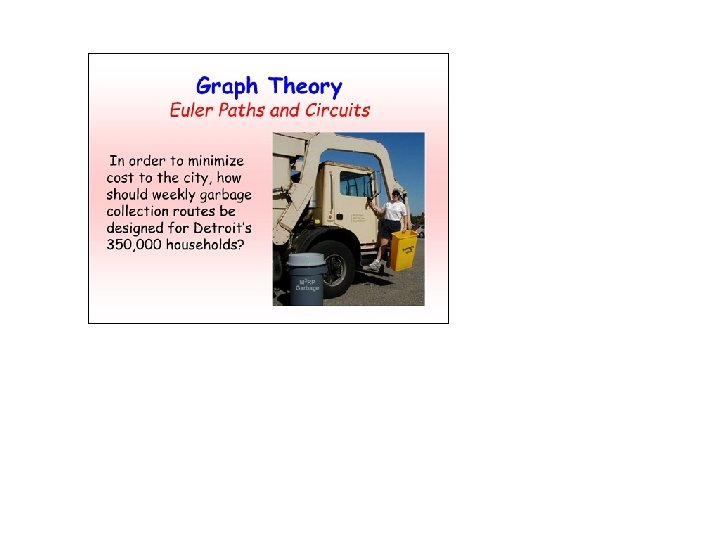Discrete Structures CSC 102 Dr Amer Rasheed COMSATS

• Slides: 52Discrete Structures (CSC 102) Dr. Amer Rasheed COMSATS Institute of Information Technology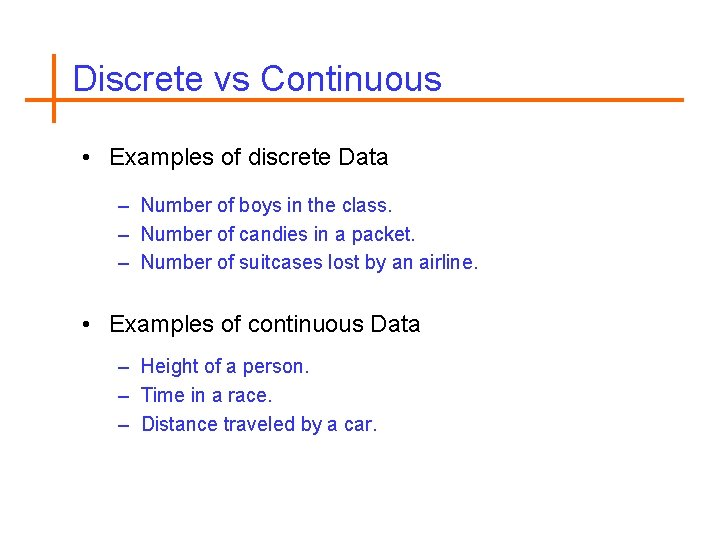Discrete vs Continuous • Examples of discrete Data – Number of boys in the class. – Number of candies in a packet. – Number of suitcases lost by an airline. • Examples of continuous Data – Height of a person. – Time in a race. – Distance traveled by a car.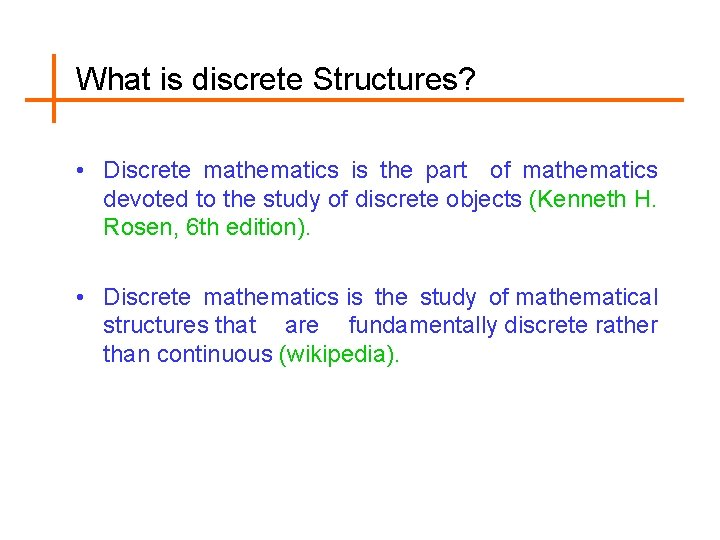What is discrete Structures? • Discrete mathematics is the part of mathematics devoted to the study of discrete objects (Kenneth H. Rosen, 6 th edition). • Discrete mathematics is the study of mathematical structures that are fundamentally discrete rather than continuous (wikipedia).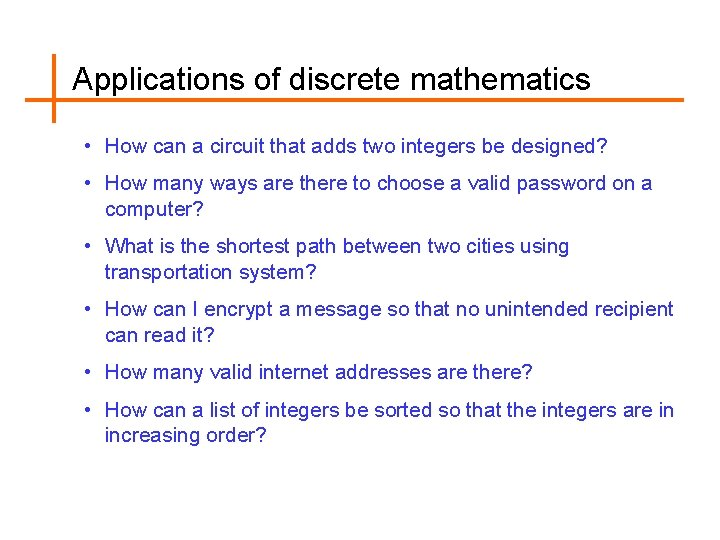Applications of discrete mathematics • How can a circuit that adds two integers be designed? • How many ways are there to choose a valid password on a computer? • What is the shortest path between two cities using transportation system? • How can I encrypt a message so that no unintended recipient can read it? • How many valid internet addresses are there? • How can a list of integers be sorted so that the integers are in increasing order?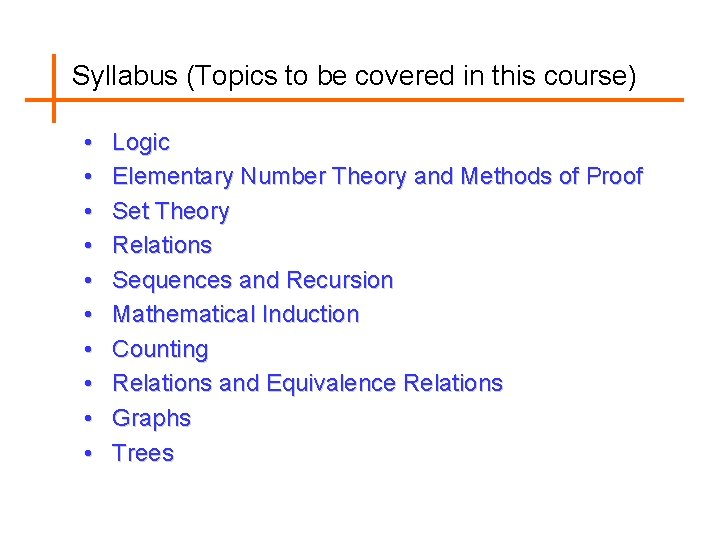Syllabus (Topics to be covered in this course) • • • Logic Elementary Number Theory and Methods of Proof Set Theory Relations Sequences and Recursion Mathematical Induction Counting Relations and Equivalence Relations Graphs TreesReference Books • Discrete Mathematics and its Applications (with Combinatorics and Graph Theory) 6 th Edition, The Mc. Graw-Hill Companies, 2007, Kenneth H. Rosen. • Discrete Mathematics with Applications 2 nd Edition, Thomson Learning, 1995, Susanna S. Epp. • Discrete Mathematics for Computer Scientists 2 nd Edition, Addison-Wesley, 1999, John Truss.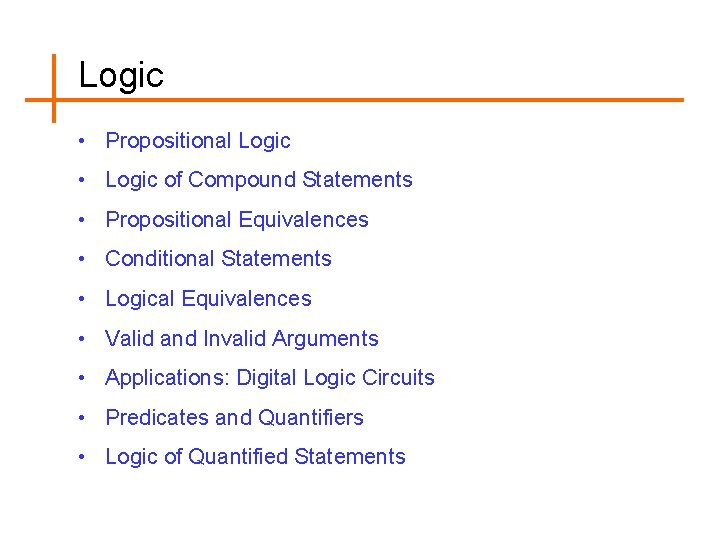Logic • Propositional Logic • Logic of Compound Statements • Propositional Equivalences • Conditional Statements • Logical Equivalences • Valid and Invalid Arguments • Applications: Digital Logic Circuits • Predicates and Quantifiers • Logic of Quantified Statements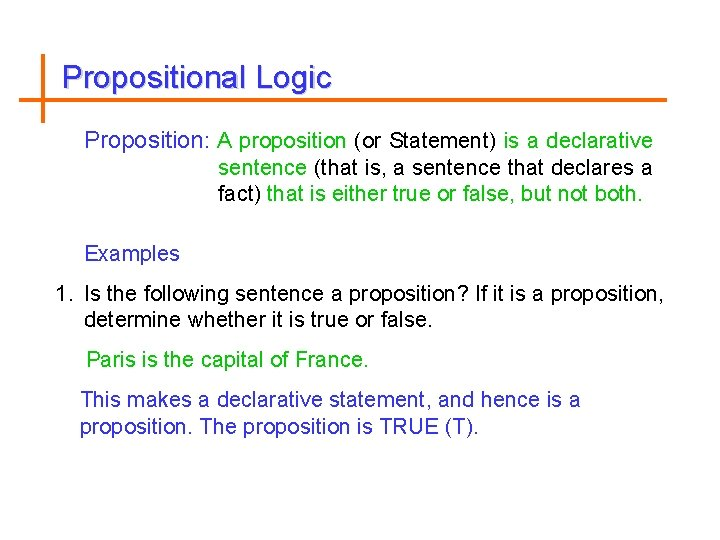Propositional Logic Proposition: A proposition (or Statement) is a declarative sentence (that is, a sentence that declares a fact) that is either true or false, but not both. Examples 1. Is the following sentence a proposition? If it is a proposition, determine whether it is true or false. Paris is the capital of France. This makes a declarative statement, and hence is a proposition. The proposition is TRUE (T).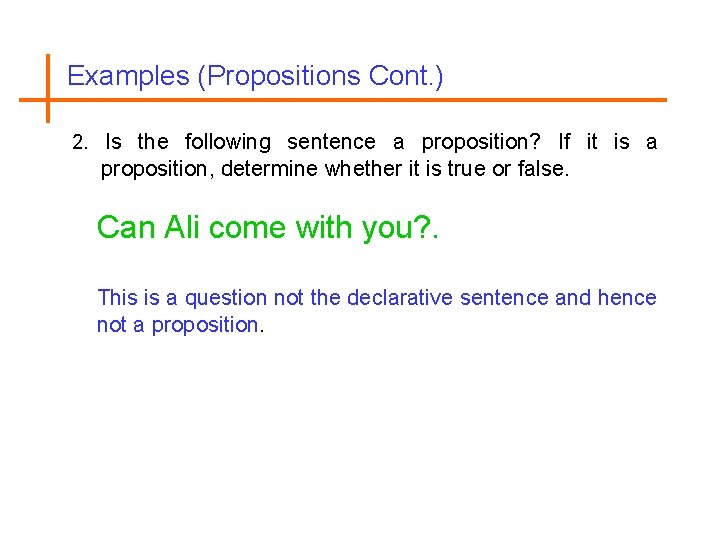Examples (Propositions Cont. ) 2. Is the following sentence a proposition? If it is a proposition, determine whether it is true or false. Can Ali come with you? . This is a question not the declarative sentence and hence not a proposition.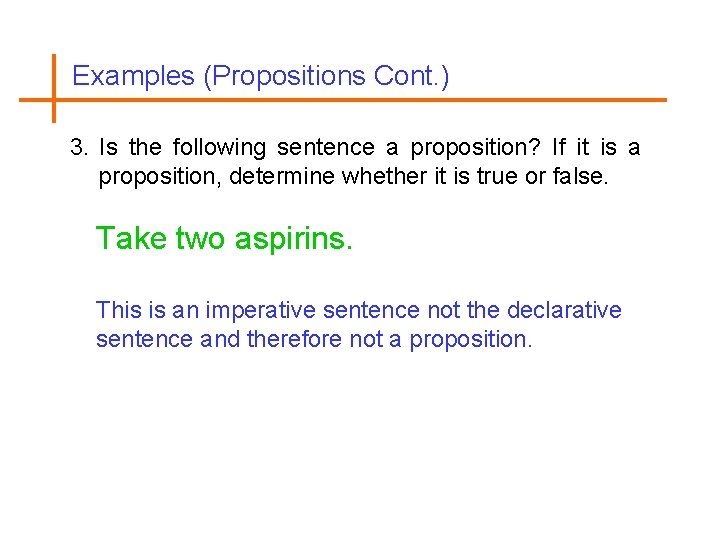Examples (Propositions Cont. ) 3. Is the following sentence a proposition? If it is a proposition, determine whether it is true or false. Take two aspirins. This is an imperative sentence not the declarative sentence and therefore not a proposition.Examples (Propositions Cont. ) 4. Is the following sentence a proposition? If it is a proposition, determine whether it is true or false. x+ 4 > 9. Because this is true for certain values of x (such as x = 6) and false for other values of x (such as x = 5), it is not a proposition.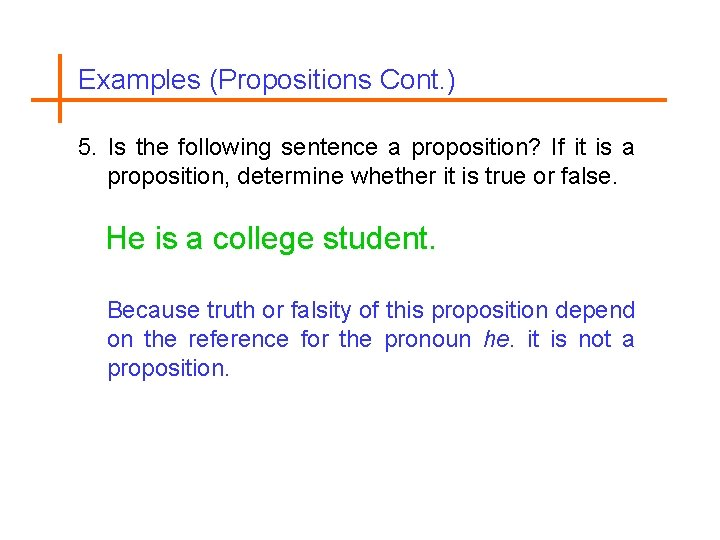Examples (Propositions Cont. ) 5. Is the following sentence a proposition? If it is a proposition, determine whether it is true or false. He is a college student. Because truth or falsity of this proposition depend on the reference for the pronoun he. it is not a proposition.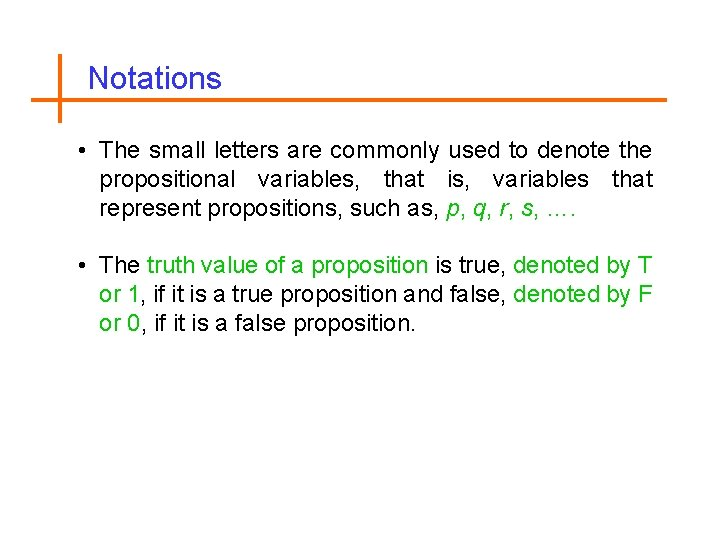Notations • The small letters are commonly used to denote the propositional variables, that is, variables that represent propositions, such as, p, q, r, s, …. • The truth value of a proposition is true, denoted by T or 1, if it is a true proposition and false, denoted by F or 0, if it is a false proposition.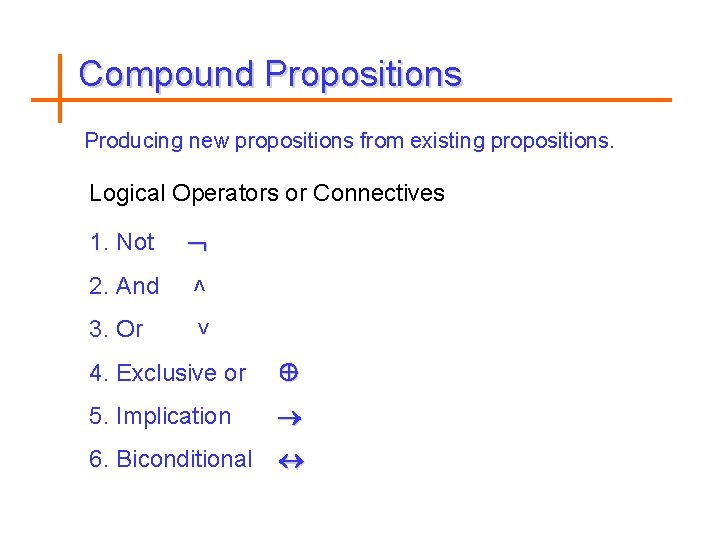Compound Propositions Producing new propositions from existing propositions. Logical Operators or Connectives 1. Not 2. And ˄ 3. Or ˅ 4. Exclusive or 5. Implication 6. Biconditional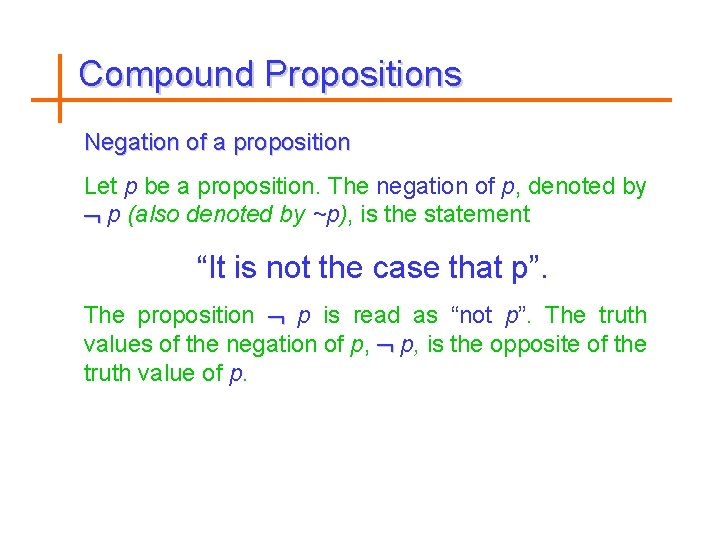Compound Propositions Negation of a proposition Let p be a proposition. The negation of p, denoted by p (also denoted by ~p), is the statement “It is not the case that p”. The proposition p is read as “not p”. The truth values of the negation of p, is the opposite of the truth value of p.Examples 1. Find the negation of the following proposition p : Today is Friday. The negation is p : It is not the case that today is Friday. This negation can be more simply expressed by p : Today is not Friday.Examples 2. Write the negation of “ 6 is negative”. The negation is “It is not the case that 6 is negative”. or “ 6 is nonnegative”.Truth Table (NOT) • Unary Operator, Symbol: p p true false true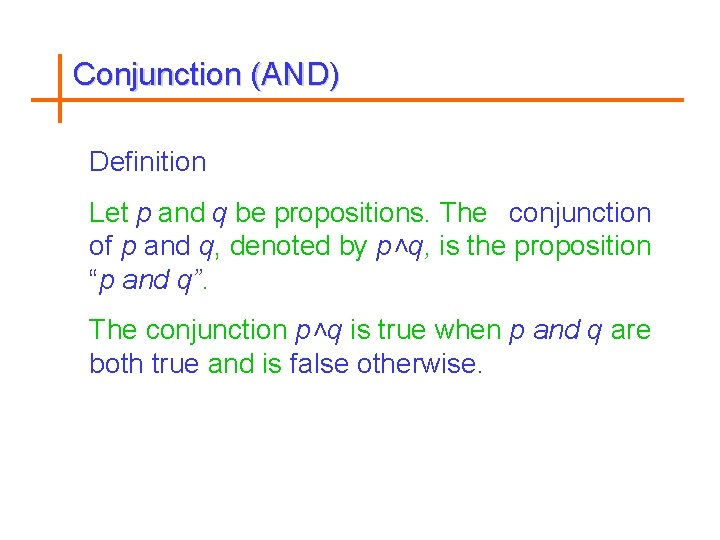Conjunction (AND) Definition Let p and q be propositions. The conjunction of p and q, denoted by p˄q, is the proposition “p and q”. The conjunction p˄q is true when p and q are both true and is false otherwise.Examples 1. Find the conjunction of the propositions p and q, where p : Today is Friday. q : It is raining today. The conjunction is p˄q : Today is Friday and it is raining today.Truth Table (AND) • Binary Operator, Symbol: p q true false true false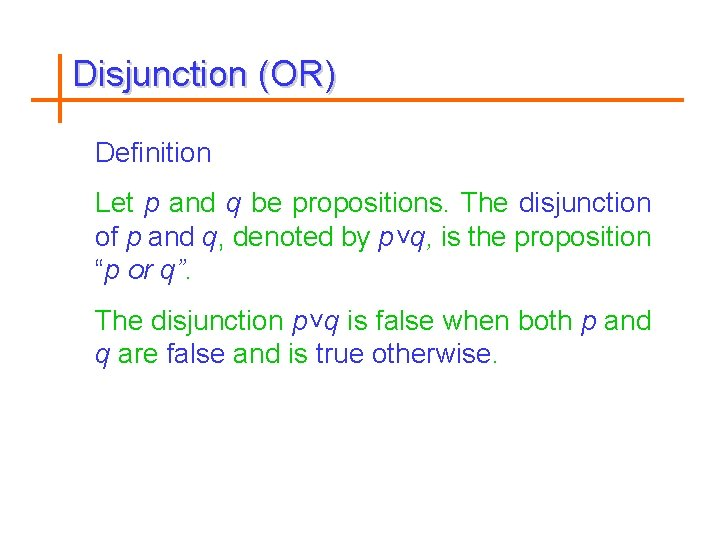Disjunction (OR) Definition Let p and q be propositions. The disjunction of p and q, denoted by p˅q, is the proposition “p or q”. The disjunction p˅q is false when both p and q are false and is true otherwise.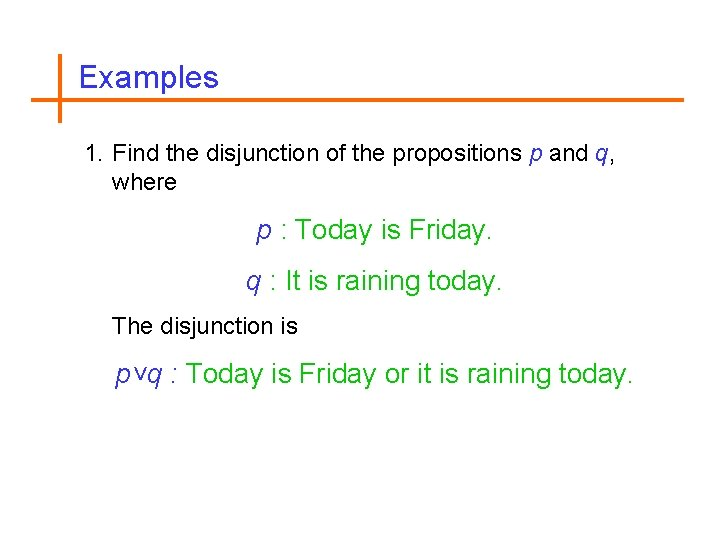Examples 1. Find the disjunction of the propositions p and q, where p : Today is Friday. q : It is raining today. The disjunction is p˅q : Today is Friday or it is raining today.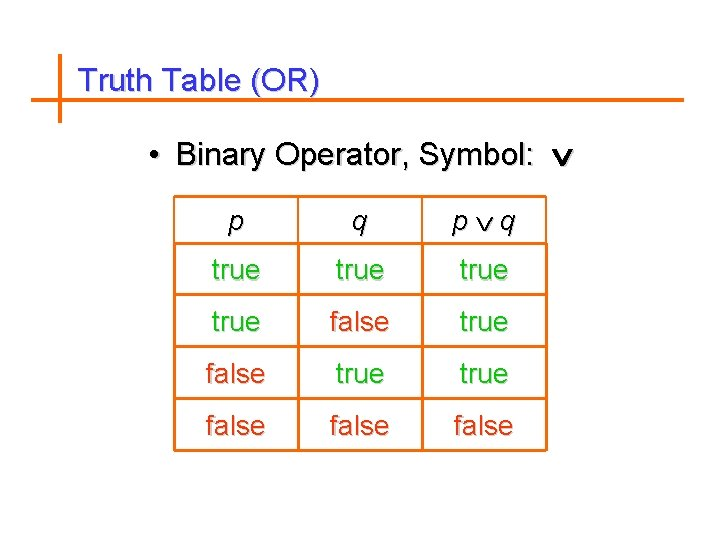Truth Table (OR) • Binary Operator, Symbol: p q p q true true false falseExclusive OR (XOR) Definition Let p and q be propositions. The exclusive or of p and q, denoted by p q, is the proposition “p q”. The exclusive or, p q, is true when exactly one of p and q is true and is false otherwise.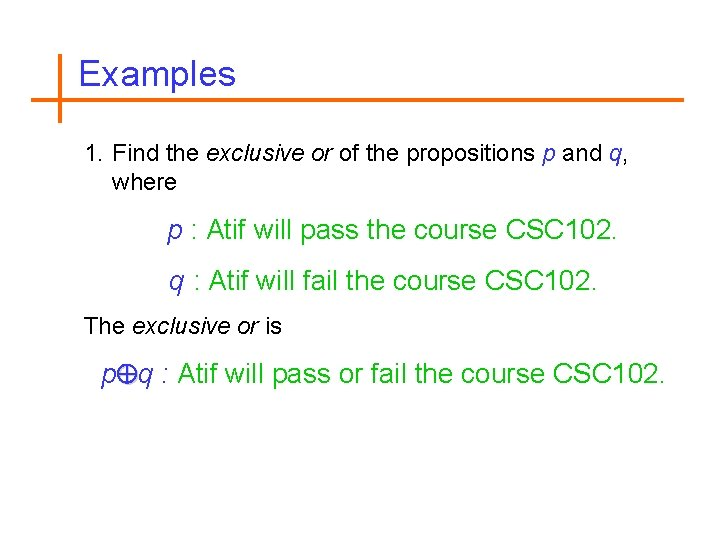Examples 1. Find the exclusive or of the propositions p and q, where p : Atif will pass the course CSC 102. q : Atif will fail the course CSC 102. The exclusive or is p q : Atif will pass or fail the course CSC 102.Truth Table (XOR) • Binary Operator, Symbol: p q true false true false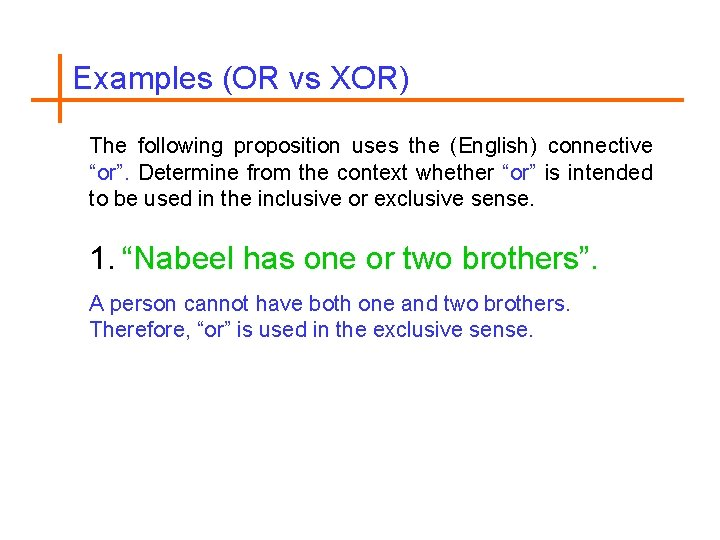Examples (OR vs XOR) The following proposition uses the (English) connective “or”. Determine from the context whether “or” is intended to be used in the inclusive or exclusive sense. 1. “Nabeel has one or two brothers”. A person cannot have both one and two brothers. Therefore, “or” is used in the exclusive sense.Examples (OR vs XOR) 2. To register for BSC you must have passed the qualifying exam or be listed as an Math major. Presumably, if you have passed the qualifying exam and are also listed as an Math major, you can still register for BCS. Therefore, “or” is inclusive.Composite Statements and operators can be combined in any way to form new statements. p q p q ( p) ( q) true false true false true false true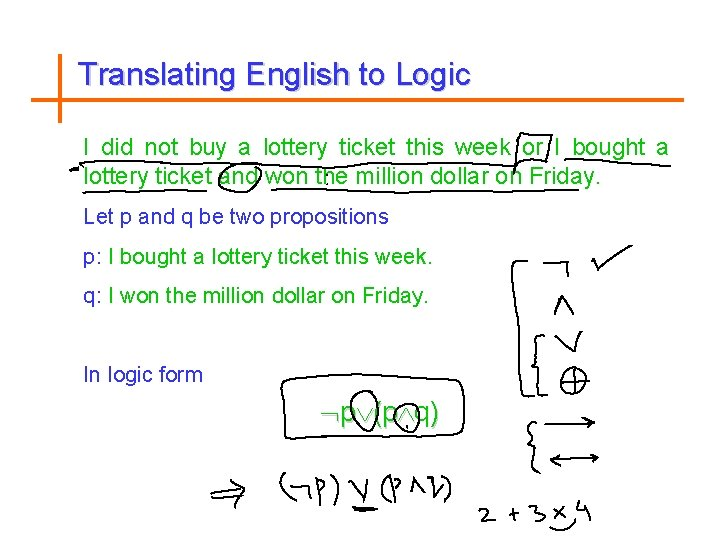Translating English to Logic I did not buy a lottery ticket this week or I bought a lottery ticket and won the million dollar on Friday. Let p and q be two propositions p: I bought a lottery ticket this week. q: I won the million dollar on Friday. In logic form p (p q)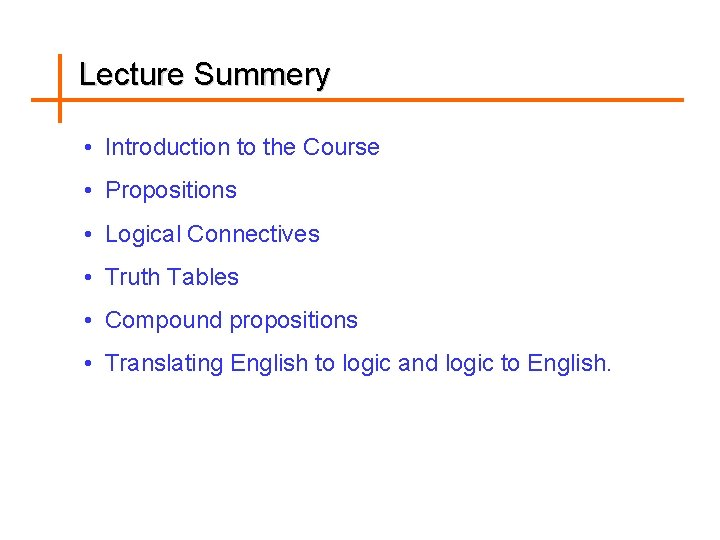Lecture Summery • Introduction to the Course • Propositions • Logical Connectives • Truth Tables • Compound propositions • Translating English to logic and logic to English.Conditional Statements Implication Definition: Let p and q be propositions. The conditional statement p q, is the proposition “If p, then q”. The conditional statement p q is false when p is true and q is false and is true otherwise. where p is called hypothesis, antecedent or premise. q is called conclusion or consequence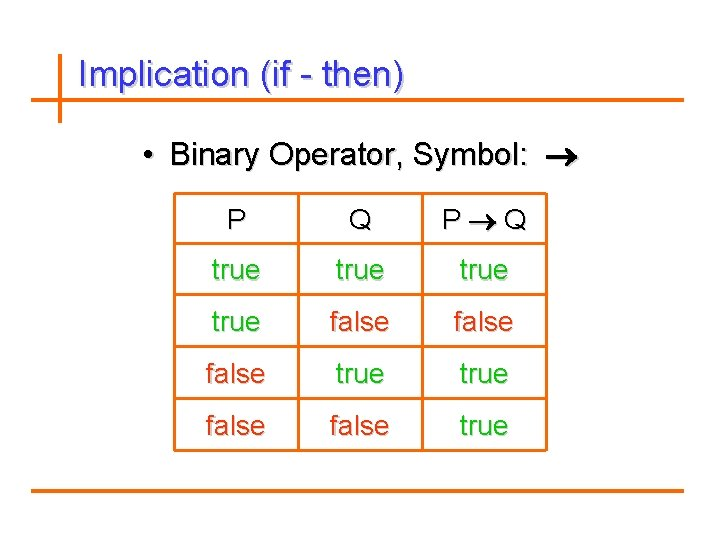Implication (if - then) • Binary Operator, Symbol: P Q P Q true false true false true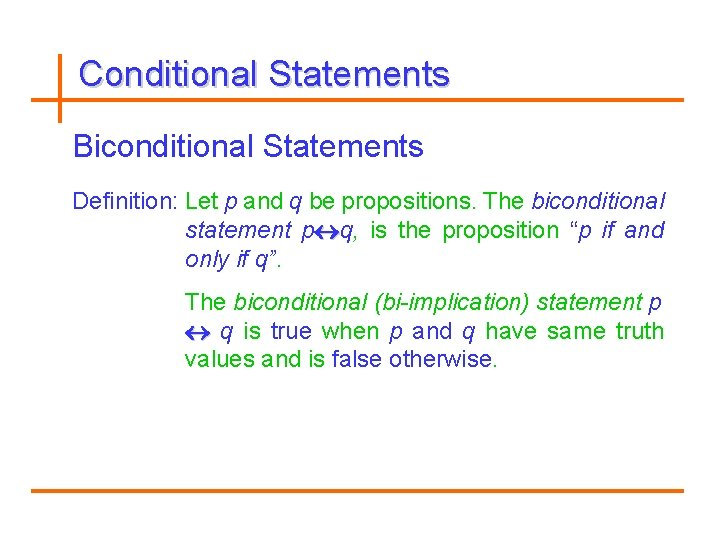Conditional Statements Biconditional Statements Definition: Let p and q be propositions. The biconditional statement p q, is the proposition “p if and only if q”. The biconditional (bi-implication) statement p q is true when p and q have same truth values and is false otherwise.Biconditional (if and only if) • Binary Operator, Symbol: P Q P Q true false false true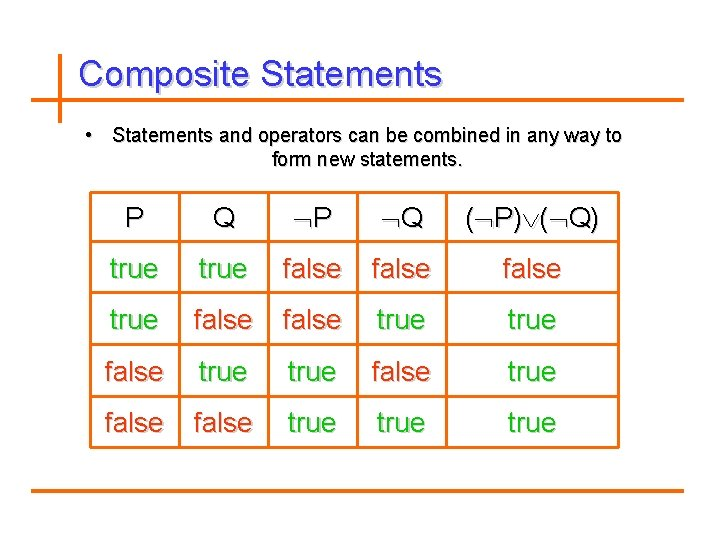Composite Statements • Statements and operators can be combined in any way to form new statements. P Q P Q ( P) ( Q) true false true false true false trueEquivalent Statements P Q (P Q) ( P) ( Q) true false true true false true • Two statements are called logically equivalent if and only if (iff) they have identical truth tables • The statements (P Q) and ( P) ( Q) are logically equivalent, because (P Q) ( P) ( Q) is always true.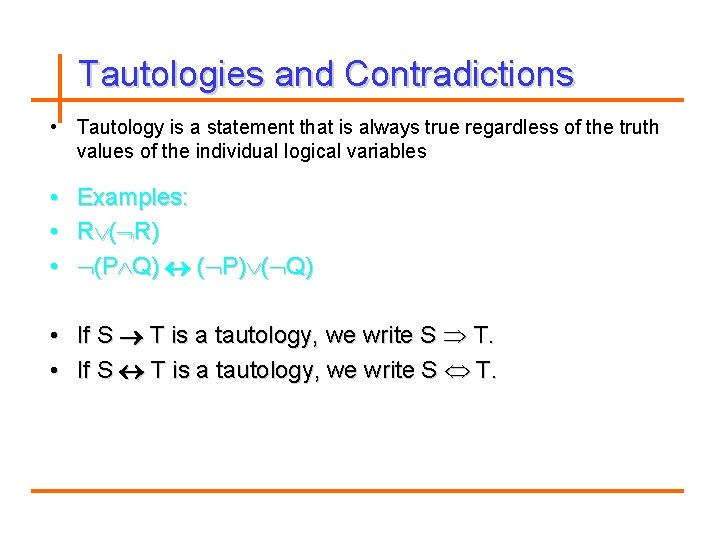Tautologies and Contradictions • Tautology is a statement that is always true regardless of the truth values of the individual logical variables • • • Examples: R ( R) (P Q) ( P) ( Q) • If S T is a tautology, we write S T.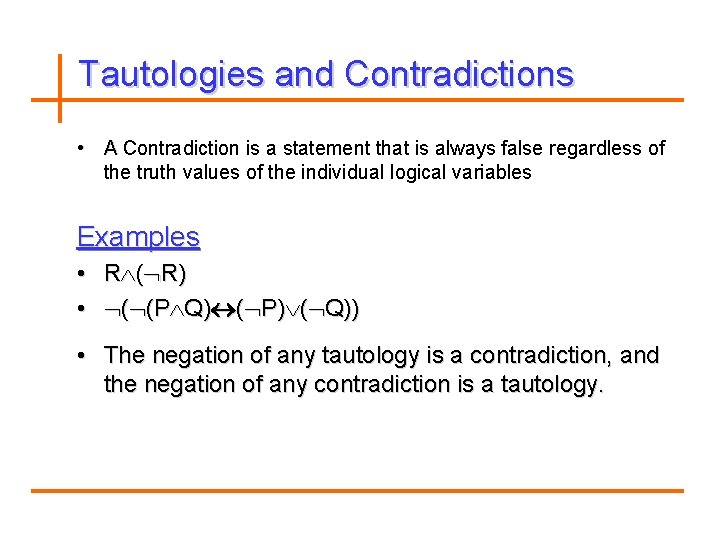Tautologies and Contradictions • A Contradiction is a statement that is always false regardless of A the truth values of the individual logical variables Examples • R ( R) • ( (P Q) ( P) ( Q)) • The negation of any tautology is a contradiction, and the negation of any contradiction is a tautology.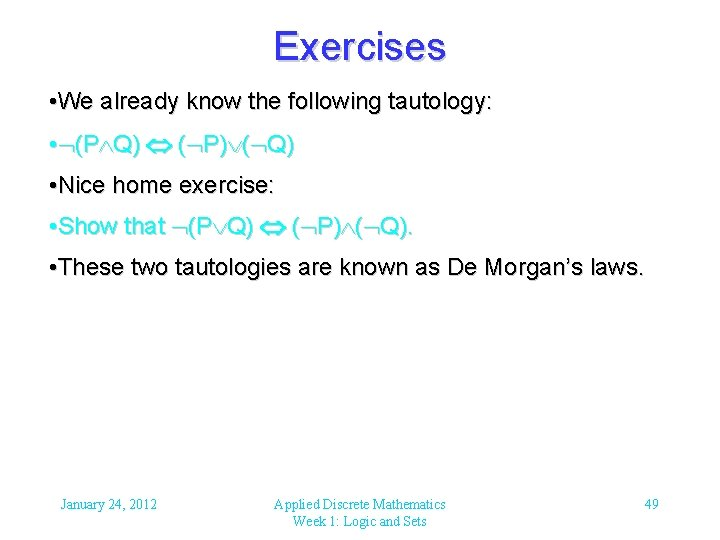Exercises • We already know the following tautology: • (P Q) ( P) ( Q) • Nice home exercise: • Show that (P Q) ( P) ( Q). • These two tautologies are known as De Morgan’s laws. January 24, 2012 Applied Discrete Mathematics Week 1: Logic and Sets 49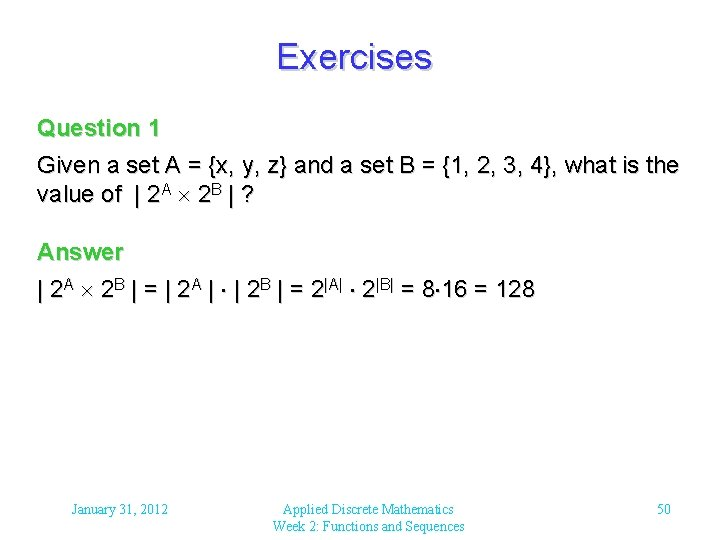Exercises Question 1 Given a set A = {x, y, z} and a set B = {1, 2, 3, 4}, what is the value of | 2 A 2 B | ? Answer | 2 A 2 B | = | 2 A | | 2 B | = 2|A| 2|B| = 8 16 = 128 January 31, 2012 Applied Discrete Mathematics Week 2: Functions and Sequences 50